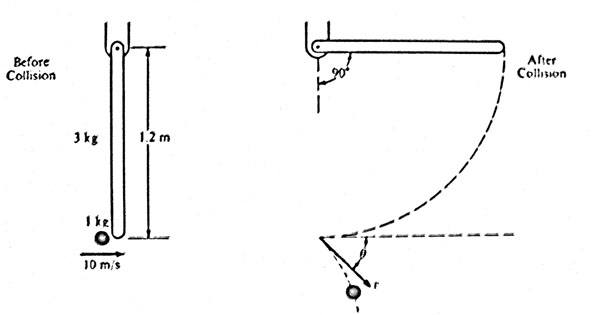# Angular Momentum - Find angular velocity of a hanging bar after a collision

I think I've gotten it but figured it would be best to get a second opinion because I feel like I made a few leaps of faith. I sincerely appreciate your time. <3

## Homework Statement

A 1.0 kilogram object is moving horizontally with a velocity of 10m/s, when it makes a collision with the lower end of a bar that is hanging vertically at rest. For the system of the bar and object, linear momentum is not conserved but kinetic energy is. The bar, of length l = 1.2m and mass m = 3kg, is pivoted about its upper end. Immediately after the collision, the object moves with speed v at an angle (theta) relative to its original direction. The bar swings freely, reaching a maximum angle of 90 degrees with respect to the vertical. The moment of inertia for the bar about the pivot is $I = (ml^2) / 3$. Ignore all friction.

Synopsis:
1.0kg object moving horizontally at 10m/s.
1.2m, 3kg bar $I = (ml^2) / 3$ suspended about its upper end.
Object hits the bottom of the bar in a glancing collision.
Bar then pivots up to 90 degrees with respect to the vertical.
Object then deflects to an angle $\theta$ below the horizontal at a velocity v.

Diagram:Questions:
1. Determine the angular velocity of the bar immediately after the collision

2. Determine the speed v of the 1-kilogram object immediately after the collision

3. Determine the magnitude of the angular momentum of the object about the pivot just before the collision

4. Determine the angle $\theta$.

## Homework Equations

The all-important: $F = ma$
Conservation of angular momentum: $L_i = L_f$
Conservation of kinetic energy: $K_o = K_ol + K_b$
Angular momentum around a point: $L = mvr sin(\theta)$
$\tau = F(lever arm) = I\alpha$
$L = I\omega$

## The Attempt at a Solution

Enclosed in quotes to make it easier to see.
1. Determine the angular velocity of the bar immediately after the collision
$$K_t + K_r = K_f + U_f$$ -- $K_t$ and $K_f$ are both zero
$$K_r = U_f$$
$$(1/2)I\omega^2 = mgh$$
$$(1/2)[(ml^2)/3]\omega^2 = 3kg * 10m/s * 1.2m/2$$ -- On the right is a leap of faith. I'm pretty sure the center of mass is what I need to account for here, and that's only moving 0.6m, so I used that.

$$[3kg * (1.2m)^2 / 6]\omega^2 = 18J$$
$$0.72\omega^2 = 18J$$

$$\omega = 5rad/s$$

2. Determine the speed v of the 1-kilogram object immediately after the collision
Total kinetic energy is conserved.
Subscript o represents object, b represents bar.

$$K_i = K_f$$ -- Initial kinetic energy equals final kinetic energy.
$$(1/2)mv^2_o = (1/2)mv^2_o + (1/2)I\omega^2$$
$$(1/2) * 1kg * (10m/s)^2 = (1/2) * 1kg * V^2_f + (1/2)[(1/3)ml^2]\omega^2$$
$$50J = (1/2)V^2_f + (1/6) ml^2\omega^2$$
$$50J - [(1/6)3kg * (1.2m)^2] * [5rad/s]^2 = (1/2)V^2_f$$
$$32m^2/s^2 = (1/2)v^2_f$$

$$v_f = 8m/s$$

3. Determine the magnitude of the angular momentum of the object about the pivot just before the collision
$$L = mvr sin(\theta)$$
$$L = 1kg * 10m/s * 1.2m * 1$$

$$L_i = 12kg m^2/s$$

4. Determine the angle $\theta$.
$$L = mvr sin(\theta)$$
$$L = 1kg * 8m/s * 1.2m * sin(\theta)$$
$$L = 9.6sin(\theta)$$

$$L_b = I\omega$$
$$L_b = [(ml^2)/3] * 5rad/s$$
$$L_b = 7.2kg m^2/s$$

$$12kg m^2/s - 7.2kg m^2/s = 4.8kg m^2/s$$ -- Plug this into the equation above...

$$4.8kg m^2/s = 9.6kg m^2/s sin(\theta)$$
$$sin(\theta) = 0.5$$

$$\theta = 30 degrees$$

Thanks so much!

Last edited: